[BACK]
 Computers, Materials & ContinuaDOI:10.32604/cmc.2022.027850Article

High-Efficiency Video Coder in Pruned Environment Using Adaptive Quantization Parameter Selection

1School of Electronics & Communication Engineering, Shri Mata Vaishno Devi University, Katra, 182320, India
2Department of Mathematics, Faculty of Science, Mansoura University, Mansoura, 35516, Egypt
3Department of Computational Mathematics, Science, and Engineering (CMSE), Michigan State University, East Lansing, 48824, MI, USA
4Electrical Engineering Department, Faculty of Engineering, Benha University, Benha, 13518, Egypt
*Corresponding Author: Krishan Kumar. Email: krishan.bpsmv@gmail.com
Received: 27 January 2022; Accepted: 08 March 2022

Abstract: The high-efficiency video coder (HEVC) is one of the most advanced techniques used in growing real-time multimedia applications today. However, they require large bandwidth for transmission through bandwidth, and bandwidth varies with different video sequences/formats. This paper proposes an adaptive information-based variable quantization matrix (AI-VQM) developed for different video formats having variable energy levels. The quantization method is adapted based on video sequence using statistical analysis, improving bit budget, quality and complexity reduction. Further, to have precise control over bit rate and quality, a multi-constraint prune algorithm is proposed in the second stage of the AI-VQM technique for pre-calculating K numbers of paths. The same should be handy to self-adapt and choose one of the K-path automatically in dynamically changing bandwidth availability as per requirement after extensive testing of the proposed algorithm in the multi-constraint environment for multiple paths and evaluating the performance based on peak signal to noise ratio (PSNR), bit-budget and time complexity for different videos a noticeable improvement in rate-distortion (RD) performance is achieved. Using the proposed AI-VQM technique, more feasible and efficient video sequences are achieved with less loss in PSNR than the variable quantization method (VQM) algorithm with approximately a rise of 10%–20% based on different video sequences/formats

Keywords: Adaptive quantization; high-efficient video coding (HEVC); quad-tree; rate-distortion optimization (RDO); video compression; variable quantization method (VQM); quantization parameter (QP)

1  Introduction

The fast-growing need for real-time multimedia applications in the field of real-time data transfer with ultra-high-definition (HD) video quality and speed has stimulated the need for high-efficiency video coding H.265/HEVC standard. ITU-T forms a Joint Collaborative Team on Video Coding (JCT-VC), ISO/IEC and Video Coding Expert Group (VCEG) in 2013 to accomplish the increased demand of HEVC standard . The development of HEVC leads to a decrease in the use of bandwidth as compared to predecessors’ techniques of video coding standards H.264/AVC (Advanced Video Coding) [4,5]. HEVC/H.265 provides a significant amount of improvement in compression and quality of video sequence for different applications such as broadcasting high-definition video data over TV and mobile channels. The computational complexity of HEVC is increased to balance between quality and compression of a video sequence. Researchers have developed several algorithms to achieve highly compressed good-quality videos with controlled complexity . In H.265/HEVC model, each portion is improved compared to the previously developed H.264/AVC coding standard, including block partitioning, transform, and quantization methods .

HEVC is frame partitioning used for code tree unit (CTU) structure forming, which replaced the Marco-block structure of its predecessors H.264/AVC. In HEVC, CTU size can vary from 16 × 16 to 64 × 64, and each CTU is partitioned into four sub-blocks . Motion estimation plays a major role in video coding and is responsible for 50% complexity of the encoders [15,16]. For controlling the complexity of encoders, a content-based coding unit partition is performed for optimizing structure, providing a large number of bits-budget and encoding time saving, improving the overall complexity of coder [17,18]. Quantization parameter played an important role in reducing complexity and maintaining the best quality with the lowest bit-budget. In previously developed video coders, the quantization parameter is set, considering the human visual system for enhancing the subjective quality of encoder output . In HEVC, two quantization schemes are considered firstly, frame-dependent quantization and secondly frame independent quantization. In the case of Frame independent, approach a standard quantization matrix used for each coding block of residual frames. In the frame-dependent approach, quantization parameters are developed according to the properties of the residual Frame, known as the adaptive quantization method . In this work bit budget control by both frame dependent adaptive quantization strategy and multi constraint pruning, combination of both techniques differentiate this algorithm from the existing algorithms. In this work, more emphasis is given to Frame dependent method. The main objective of work to develop a common method which is suitable for fast motion and slow motion video sequence. Another goal of this work is to reduce the bit budget of video sequence without loss in quality. The paper is organized as follows. First, the literature review is discussed in Section 2. Then Section 3 presents the proposed method. In Section 4, the experimental results are shown. Finally, the conclusion is discussed in Section 5.

2  Literature Review

The adaptive quantization method using 35 intra-prediction angles and the size of transform units (TU’s) in HEVC is discussed by the author . In this method, the user has more flexibility regarding the quantization parameter selection, but it applies only to the residuals obtained in intra-prediction mode. For further enhancement in the subjective quality of the HEVC encoder, a block-level adaptive quantization method is used. The author proposed an adaptive block-level quantization method to quantize each type of block individually by reducing bit budget and complexity . There are numerous coding tools for enhancing the coding efficiency of HEVC among all recent transform coding technique increases the efficiency of HEVC with variable block sizes . Quantization parameter directly varies with the residual signal. N-level quantization coefficient is transformed at N-levels, and this N-level quantization is performed according to the scanning order of coefficients . By soft thresholding approach, quantization transformation of variable blocks is done with energy and improved reconstructed signal efficiency . The performance of HEVC coding is improved by the structure similarity index-based quantization method . The author  proposes an adaptive quantization method for HEVC based on the previously encoded residual block’s statistically computed data.

Many researchers purposed different rate distortion (RD) optimization techniques with adaptive quantization methods to reduce bit-budget with low complexity. RD optimization techniques modify the rate-distortion coefficients so that a targeted bit-budget with reduced system complexity is achieved [29,30]. Authors proposed [31,32] an idea to compute the RD cost of quantized coefficients in place of real coefficients for reducing the heavy load of computation. Further, to improve rate-distortion optimization, quantization up to a level can be done using the encoder’s RD optimization technique complexity. Adversely from the existing literature survey on quantization parameter effect on the quality and bit budget control, the present work provides a method of reducing complexity without any loss in quality . The proposed adaptive information-based variable quantization method (AI-VQM) is integrated with a multi-constraint pruning algorithm, reducing the paths from a set of pre-computed paths and reducing complexity by maintaining the quality of the reconstructed video frame. In Multi constraint based pruning only those paths are selected which satisfied the rate and distortion condition and other one is rejected. Paths which satisfied the both condition in single path provides quality of service in next generation network. Our proposed method depends upon the compression factor, and the compression factor varies with input video sequences. Using this compression factor variation strategy, the purposed method is more suitable for a different class of variable resolution video sequences. The proposed algorithm using constrained-based pruned algorithms reduces complexity to control RD cost compared to traditional RD optimization.

3  Proposed Work

With the development of H.264/AVC, HEVC with different parameters played an appropriate role in the encoding process of video streams like minimum and maximum size of coding unit, level of frame partition, quantization parameter, search range frame rate and motion estimation technique to control the bit-budget of input video sequences. In H.265/HEVC, the coding tree unit (CTU) is the core unit layer; this core unit’s size is always larger than the basic coding layer unit called macroblocks in the previous encoder. The large size of the coding unit is responsible for the complex structure of the quadtree and increases the encoder’s complexity. To control the encoder’s complexity, numerous researches are carried out in this stage, pruning of quadtree is required for saving more bits. There are several pruning techniques adopted for saving a bit of budget with some acceptable amount in degradation in quality in several research articles.

In this study, a considerable number of efforts have been made to reduce the encoder’s complexity by developing a smart quadtree structure using soft computing techniques. This smart quadtree reduces the encoder’s complexity by stopping the irrelevant information at an early stage and transmitting the relevant information for further processing during tree formation improves the quality and bit budget. A modified quadtree structure gives more precise information about the edges than the previous quadtree formation strategy. This modification in HEVC architecture provides a suitable enhancement in performance parameters. This smart quadtree structure strategy is introduced in modified HEVC architecture, as shown in Fig. 1. Among different coding steps of HEVC, inter prediction is most computation concentrated due to the presence of motion estimation and compensation part. The goal of motion estimation is to search for candidate blocks in a previously decoded Frame with a block matching algorithm. These algorithms are responsible for motion vectors by extracting motion activity from the input video frame  and providing residual error information depending upon the number of motion vectors generated during motion estimation and compensation. In the modified architecture of HEVC, an exhaustive motion estimation search algorithm is used to find more precise motion information by using a motion vector. In this modified architecture multi constraint pruning play a key role to control the bit budget of reconstructed video sequence. This pruning technique prune the paths which does not satisfy the multi constraint condition.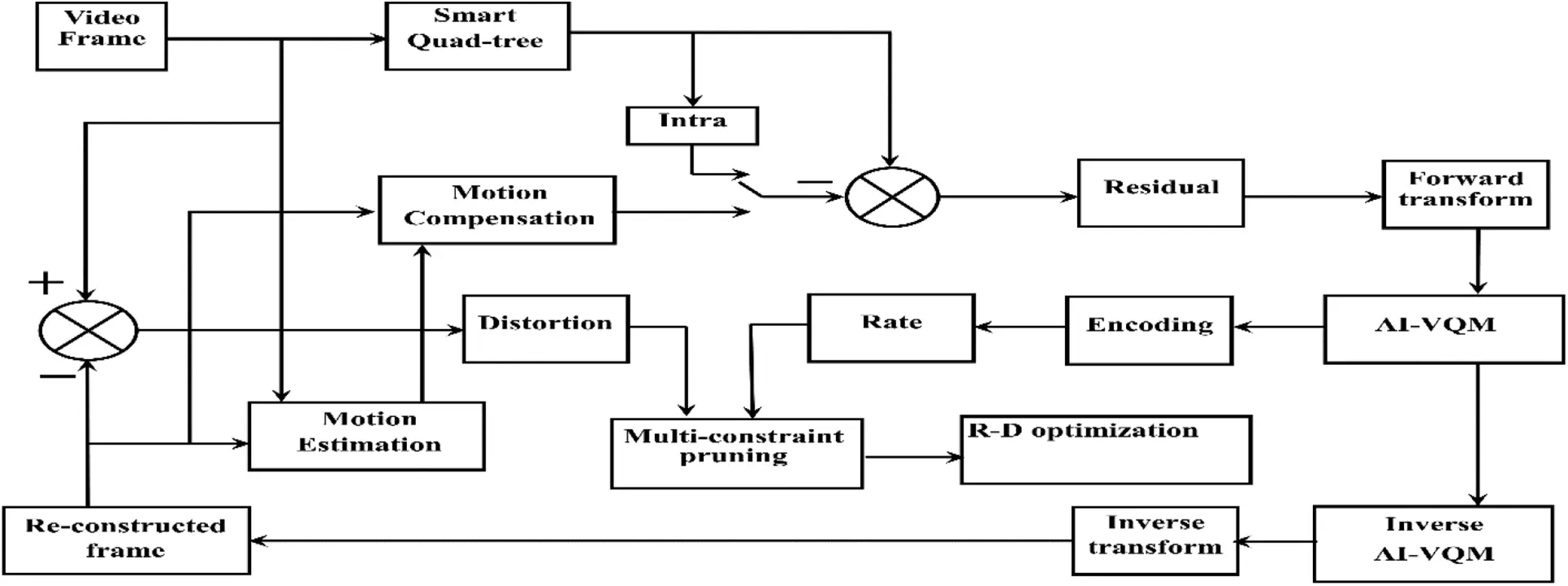Figure 1: The modified architecture of HEVC using the proposed AI-VQM technique and multi-constraint prune algorithm [1,2]

Similarly, the quantization parameter (QP) plays a major role in saving a large bit of budget for controlling residual information. High quality and a smaller number of bits saving in the opposite high value of quantization parameter are responsible for more bits saving and some degradation in quality  achieved due to low value of QP. Degradation in quality is avoided using variable quantization (VQM), which provides a low value quantization parameter to high information regions and vice-versa. Anyhow computational complexity is increased to find the optimum value of quantization parameter for different classes of a video sequence. An AI-VQM technique is inducted in the standard architecture of HEVC, as shown in Fig. 1, to avoid computational complexity. Adaptive quantization is classified into two types, i.e., forward and backward adaptation. Input video sequences are adaptatively adjusted according to the quantization parameter in forwarding adaptation.

On the other hand-coded output of the system is adjusted according to the quantization parameter in backward adaption. Comprehensively it is stated that adaptive quantization means to adjust the quantization parameter dynamically with variable classes of video sequences [22,23]. In the proposed work AI-VQM method, a compression factor is calculated depending upon the current Frame and reconstructed Frame. Compression factor adaptively changes with different classes of video sequences. A small value of compression factor is adaptively adjusted for the high motion activity portion, and a large value is adjusted for the motionless portion of the video frame. Overall, the suppression of information level depends upon the dynamic compression factor.

3.1 Model of VQM

In the VQM block level quantization parameter method, different quantization parameters are fixed for each type of block for a video sequence. Information level of blocks may vary according to a different class of video sequences, and each class of video sequences required a new set of quantization parameters.

It is necessary to understand the bit budget requirement for the VQM technique to analyze the block-level quantization method. However, the rate (R) and distortion (D) bits are responsible for the total budget for video sequences. A generalized rate bit budget can be expressed by Eq. (1).

R=n×mln2((1er)ln(1er)+rsinhrerln(sinhr))(1)

where,

m×n Video frame dimension,

r = rate constant

The predicted rate is a function of ‘r’ and can be expressed as Eq. (2)

r=K×2(QP4)6MSEπ(2)

where K is the total number of coefficients in a macroblock or CU. From Eq. (1), it is observed that ‘R’ appears more sensitive to a quantization parameter (QP) as compared to mean-square-error (MSE). In the block-based quantization method, a different quantization parameter is used for different types of blocks. Using the quadtree strategy, it is found that the small size of blocks having more information as compared to the large size of blocks. In the considering of the above strategy Eq. (2) can be rewritten for the block-based quantization method using a different value of quantization parameter for different size of blocks as follows:

r64×64=K64×64×2(QP64×644)/6MSE64×64π(3)

r32×32=K32×32×2(QP32×324)/6MSE32×32π(4)

r16×16=K16×16×2(QP16×164)/6MSE1616π(5)

r8×8r8×8=K8×8×2(QP8×84)/6MSE8×8π(6)

r4×4=K4×4×2(QP4×44)/6MSE4×4π(7)

For block-level quantization method, rate function ‘r’ in Eq. (2) can be rewritten as

rVQM=r64×64+r32×32+r16×16+r8×8+r4×4(8)

Putting the value of rVQM in Eq. (1) and rate equation is rewritten as

RVQM=n×mln2((1erVQM)ln(1erVQM)+rVQMsinhrVQMerVQMln(sinhrVQM))(9)

The cost function of the block-level quantization method (JVQM) can be expressed as

JVQM(cost)=DVQM+λRVQM(10)

where cost is calculated with the help of cost function JVQM in variable quantization method.

In the above block-level quantization method, the system’s complexity is increased for finding out the optimal value of the quantization parameter for each type of block size. This optimum value is not suitable for each class of video sequences because each type of blocks’ information level is changed with video sequences and again finds out the optimum value of quantization parameter for a different video sequence. To avoid finding out the optimal value of the quantization parameter, an adaptive information-based quantization method is developed to meet the requirement of various video sequences.

3.2 Proposed Model of AI-VQM Technique

A quality control algorithm has been implemented in the proposed model for high-resolution video transmission. The main focus for this advanced modification in the HEVC encoder is to reduce complexity with large bit saving and maintaining the objective and subjective quality of the reconstructed video sequence. In HEVC structure, better video quality is achieved using efficient utilization of soft computing based quadtree structure combined with adaptive information based variable quantization method (AI-VQM) strategy in a multi-constraint prune environment. In soft computing-based partitioning of the Frame, the low-frequency region is determined with more granularity than the high-frequency region and forms an advanced smart quadtree structure. We divided the largest coding unit into small-small units based on edge information on coding tree blocks in the quadtree decomposition method. If edge information on residual prediction blocks (Eri) is greater than the pre-computed threshold value (Eth), then by using the soft computing method, the coding unit (CU) blocks are further considered as prediction units (PU) and transform units (TU) otherwise same CU is consider as a leaf of the tree representation. Similarly, the same methodology is applied to 4 × 4, 8 × 8, 16 × 16 and 32 × 32 size, and the complete tree is formed so that a wide range of quadtree depth exists as compared to H.264/AVC [4,5]. In our proposed work, threshold value Eth adaptively fixed according to video sequence using soft computing technique and decided that whether CU is further spitted or not.

Er (32×32)=1m×ni=0mj=0nri,j2(11)

If Eth < Eri condition is satisfied, then E is further divided into the Er(16×16) and so on. By adopting this method, an optimized graph is formed with reduced complexity and bit budget. Bit budget can be further reduced by using motion estimation algorithms and to find out the motion activity in a predicted frame to construct the residual Frame. The residual energy of residual Frame of few low frequencies and high-frequency coefficients is applied using discrete cosine transform (DCT) in Transform Unit (TU). These transformed coefficients have large magnitude coefficients in the low-frequency region and small magnitude coefficients in the high-frequency region. Therefore, more information is concentrated in the low-frequency region as compared to the high-frequency region. As discussed above, in quadtree formation, the high-frequency region represents a large block, and the low-frequency region represented the block’s small size.

Further control in bit budget and complexity, AI-VQM technique is the used-on basis of information levels of blocks which varies according to the resolution of the video stream. The proposed method forward adaptation method is used at the encoder side, and simultaneously adaptation coefficients are transferred to the decoder side to reconstruct the original sequence. There are several methods used to perform the adaptive quantization method in video compression. In this method moving and stationary portion of the input video sequence is analysis on the basis of variance and both portion is quantized accordingly. This methodology provides the high quality and large bit budget saving. This work variance-dependent adaptive quantization method is used in a multi-constraint environment for video stream compression due to its low-frequency pass nature, based on a statistical analysis of variance. Variance analysis of input sequence can be expressed as Eq. (12).

VC=1Ni=1N(xixi¯)2(12)

where xi is the pixel value at ith position, xi¯ is the mean value of all the pixels in the block, and ‘N’ is the total number of pixels in the blocks, and ‘V’ represents the variance of the block. Similarly, variance output of current block and reconstructed blocks is represented in Eqs. (13) and (14).

VC=1NCi=1NC(xicxic¯)2(13)

VR=1NRi=1NR(xiRxiR¯)2(14)

Residual signal Eri is represented by the variance difference of current and reconstructed blocks shown in Eq. (15).

Eri=1NCi=1NC(xicxic¯)21NRi=1NR(xiRxiR¯)2(15)

The compression factor (CF) calculated according to Eq. (16) using Eqs. (13) and (14).

CF=VCVRmax(VC,VR)(16)

Vc is the variance of the current block, and VR is the variance of the reconstructed block. The compression factor varies with the variance of the blocks of a different class of video sequence. Variance output provides smoothness in the signal because it provides the low-frequency coefficients at the output side.

In comparing the block-level quantization method in the variance-dependent method, there is no need to find out quantization parameters for different types of video sequences. In variance dependent method, residual error signal varies according to computed compression factor, and this compression factor varies with the different classes of video sequences. It is observed that value of quantization parameter varies accordingly with compression factor. So that more will be the value of compression factor means more smoothness and high quantization factor is required and vice versa.

JAIVQM(cost)=DAIVQM+λRAIVQM(17)

JAIVQM(cost)<JVQM(cost)(18)

From the above observation, the proposed adaptive quantization method’s cost function is always less than the variable quantization method and is represented by Eq. (18).

3.3 AI-VQM Technique in Multi-Constraint Pruned Environment

To further control this computation and bit budget requirement, A* prune algorithm is proposed along with the above method and pruning the pre-computed shortest paths based on multi constraints parameters. At this stage, the problem becomes the constraint-based, and we convert this constraint-based problem into unconstraint problem using Lagrangian optimization techniques. In Lagrangian optimization, at a particular value of Lagrangian coefficient gives the best optimum paths based on bit budget.

Overall in our proposed work efforts are taken to the optimization of selected paths with the help of an AI-VQM technique by suppression of non-informative portion as compare to informative portion and developed high resolution and highly bit saving algorithm. Finally, algorithm output provides k-optimized paths having different bit budget under multi constraint environment. These multi constraint-based multiple paths fulfil the demands of user in different domain of video compression. A comparative analysis is done between AI-VQM based multiple paths and VQM based multiple paths in the multi-constraint environment.

Based on the bit budget, a closed loop graph G = (N, E) is formed with multiple paths from source (S) node to the destination node (D). In this graph ‘N’ will be set of nodes and ‘E’ will be the set of links and each link (i, j) belongs to ‘E’ associated with quality of service parameter.

In multi constraint shortest path (MCSP) problem k-shortest paths are found out based on length of the path, sometime this length called the cost of the path from the source node (s) to the destination node (D). If K is number of paths in the network and ‘Cr’ is the number of constraints associated with each path, MCSP written as

Wr(p(S,D))=(i,j)p(S,D)Wr(i,j)Cr(S,D)(19)

where r=1,2,3R.

Now A* search combines with proper pruning technique and to get A* prune algorithm which is most suitable for solving the MCSP problem. This MCSP under modified A* Prune algorithm grows a candidate path list in such a manner the most likely shortest feasible paths extended first. Extended paths that violate the constraints condition are pruned from the candidate list. This pruning strategy of A* algorithm reduces the complexity and bit budget of the proposed algorithm. In A* prune algorithm minimum cost path is find, those satisfy the constraints condition. This algorithm provides best results under more than one constraints condition. It is observed that this algorithm will be optimal and complete.

3.4 Rate-Distortion Analysis Using AI-VQM

R-D cost plays a major role inappropriate mode decisions in HEVC. The appropriate mode is the good combination of bit budget of rate and the distortion in the path. The video sequence’s Bit budget is calculated after passing the sequence through transform, quantization, and entropy coding stage, similarly distortion estimated with the difference of current Frame, and reconstructed Frame using a feedback loop. By reducing the computation complexity of the encoder, increases the data transmission speed of the encoder.

Therefore, RD cost depends on the information present in the particular Frame and simultaneously optimizes the different HEVC coder parameters.

In the proposed work, a considerable amount of effort has been done to reduce the RD cost using a modified A* Prune algorithm with the AI-VQM technique. The proposed algorithm using AI-VQM reduced the bit budget for residual information and simultaneously pruning algorithm prunes infeasible partial paths at an early stage, which are not satisfied with the multi-constraint condition. The aggressive pruning, modified in the architecture of the HEVC, explores the fast network and excellent saving in the big-budget, which is shown in Fig. 1. Further, using Lagrangian optimization provides the best combination of the rate and distortion and provides the rate-distortion optimized multi constraint-based video sequences. In this work beauty of lagrangian function is to satisfy the both rate and distortion condition with achieve the less bit budget objective of work. Finally, this work’s outcomes provide enormous savings in bit budget and maintain the quality of the transmitted video sequence. Simulation of different resolution video sequences using AI-VQM and pruning technique provides various multiple paths for multiple video compression applications.

4  Result and Discussion

To evaluate the performance of the proposed AI-VQM algorithm, we test it on the latest encoder H.265/HEVC and simulate using MATLAB software. Three different classes of video sequences with different resolutions and characteristics are tested on the same encoder with the proposed algorithm. Variable quantization method (VQM) configuration, and proposed AI-VQM configuration approaches are tested and compared using MATLAB simulation.

Rate-distortion performance is evaluated in terms of bit budget and PSNR where the negative sign for bit budget and a positive sign for PSNR represent performance gains over the VQM algorithm. From the Tab. 1, it can be seen that AI-VQM method achieve −24.04% bit-budget and −1.48% time saving on average as compared with the standard VQM, method and 3.45 dB PSNR gain in case of high definition park joy video sequence with resolution 3840 × 2160 pixel. It can be seen from above Tab. 1 that proposed algorithm outperform as compare to standard VQM at different motion activity of video sequences. Tab. 2 shows the results of crowd run high definition video sequences having a resolution of 1920 × 1080 pixel. In this table, the proposed method achieves −30.61% average bit budget and −6.79% time-saving, 2.96 dB average PSNR gain as compare to VQM.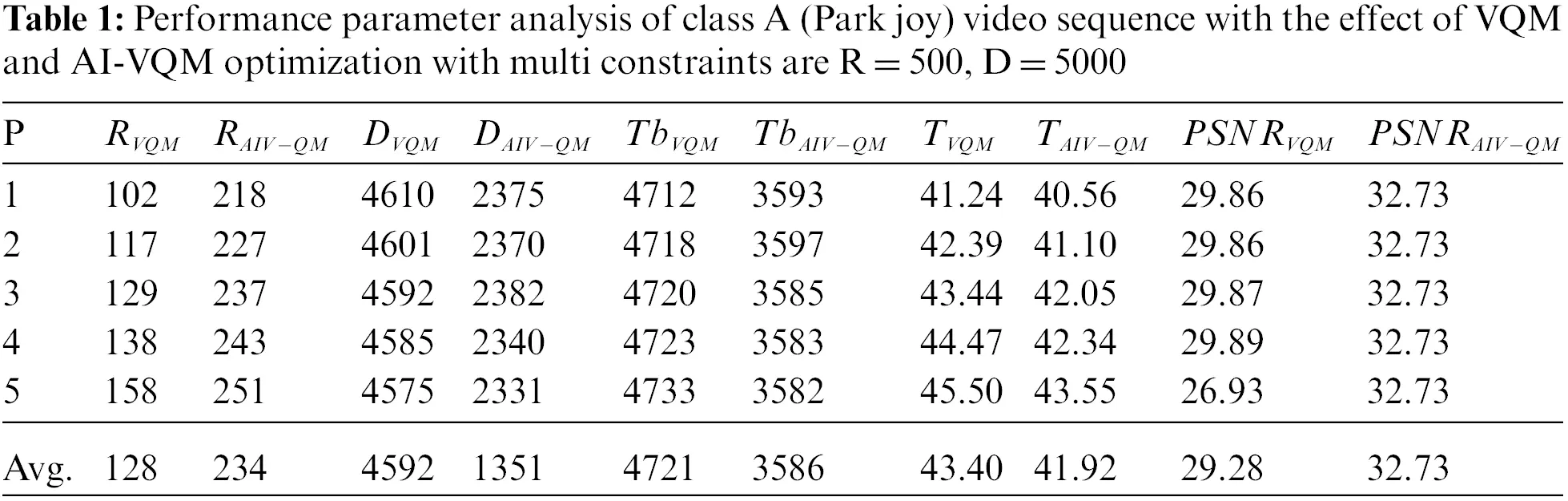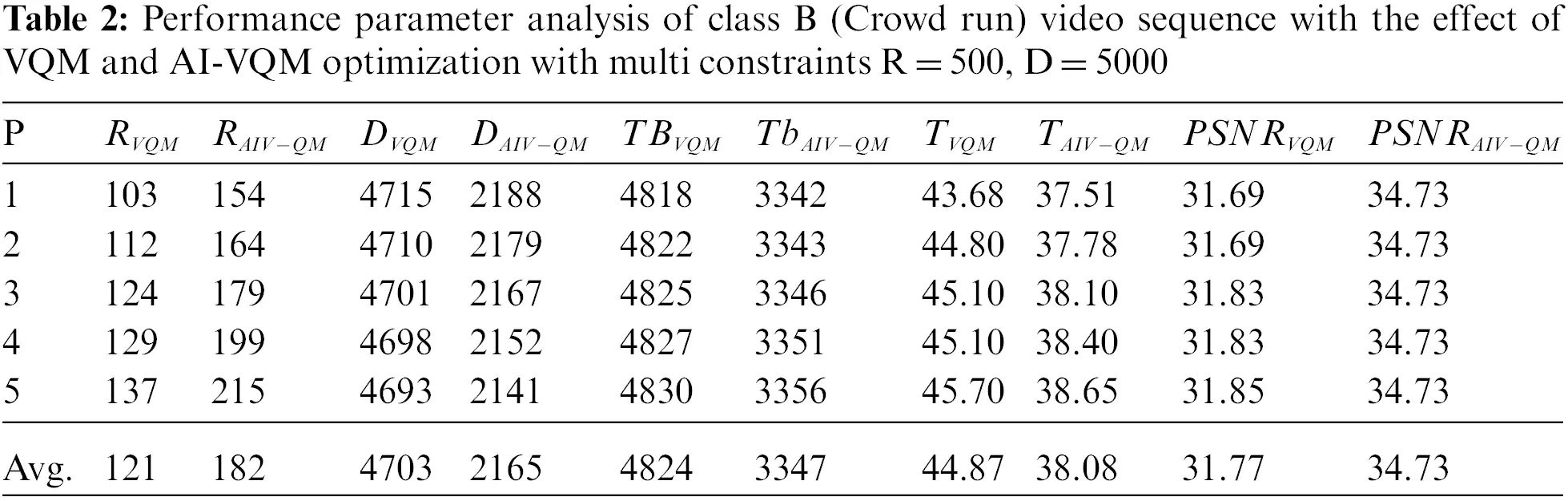Tab. 3 shows the results of the old city for a mobile domain with resolution 1280 × 720 p. It is observed that the proposed method achieve −34.27% bit budget and −2.1% time saving with 1.34 dB improvement in PSNR gain. To better understanding the behaviour of the proposed algorithm, it is necessary to understand the criteria of selection of quantization parameters. In the case of the VQM algorithm, it is a complex task to calculate the quantization parameter for a different class of video sequences. In the case of the proposed method, a non-informative portion of the video sequence suppressed according to the compression factor, which varies according to the variance of a different class of video sequences. Multi-constrained pruned algorithm and smart quadtree reduced the time complexity of the proposed AI-VQM algorithm as compared to VQM algorithm. Form Tabs. 13, it is observed that the proposed algorithm gives improved results for different resolution video sequences. In the proposed algorithm with the use of the smart quadtree, motion activity region find out more precisely and assign more bits to this region as compared to VQM algorithm and enhanced the PSNR value. Figs. 1, 3 and 5 shows the quadtree structure using the variable quantization method (VQM) and Figs. 2, 4 and 6, 7 shows the quadtree structure using the proposed AI-VQM technique. From the two sets of the quadtree structure, it is concluded that in the case of AI-VQM motion activity is find out in a more granular way by forming the quadtree structure up to a high level. A higher level of quadtree formation increased the bit budget, to overcome this problem proposed algorithm integrated into multi constraint pruned environment and reduce the bit budget. In HEVC levels of quad tree structure is increased, by this motion activity in video frame found more accurately. It is observed that if more number of bit budget allocate to the motion activity good quality of output video sequence achieved. It is also observed that if there is number of levels is increased, complexity of the system is increased. To reduce the complexity of purposed algorithm, MCSP used with A*Prune algorithm and pruned the paths that violate the constraint condition.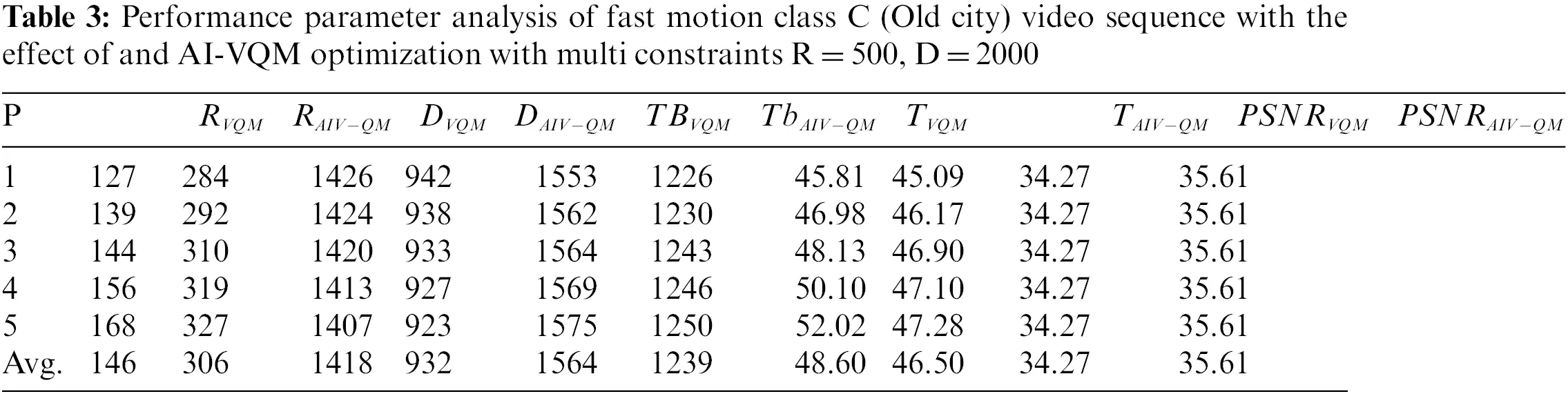Figure 2: Original and reconstructed frame with quadtree structure of optimal path (bit budget, PSNR) using VQM algorithm for HD video sequences of pak joy

All tests were run on a 2016 Laptop with a Quad-Core i3-2.60 GHz CPU and 4 GB memory.Figure 3: Original and reconstructed frame with quadtree structure of optimal path (bit budget, PSNR) using AI-VQM algorithm for HD video sequences park joy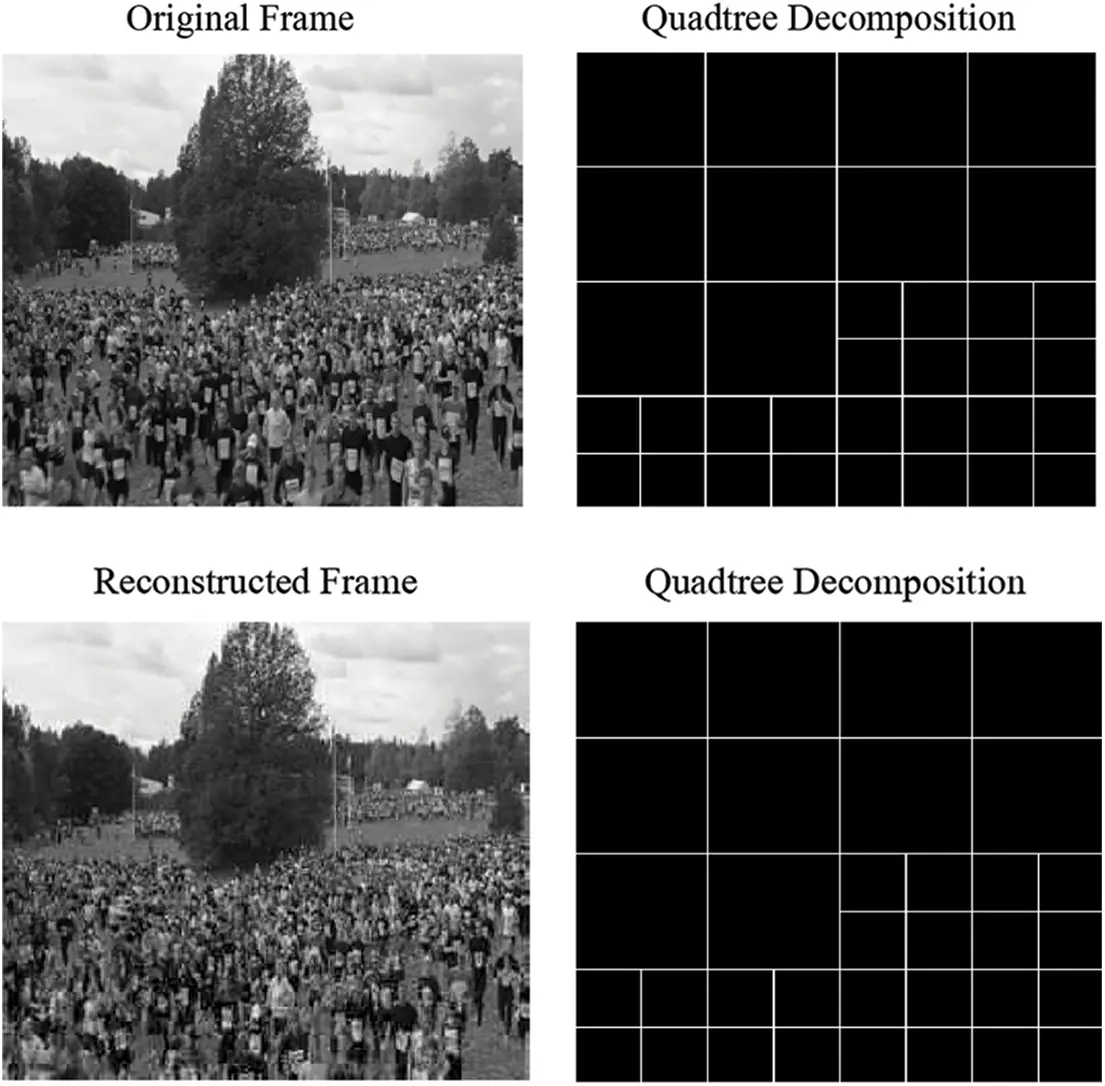Figure 4: Original and reconstructed frame with quadtree structure of optimal path (bit budget, PSNR) using VQM algorithm for HD video sequences of crowd run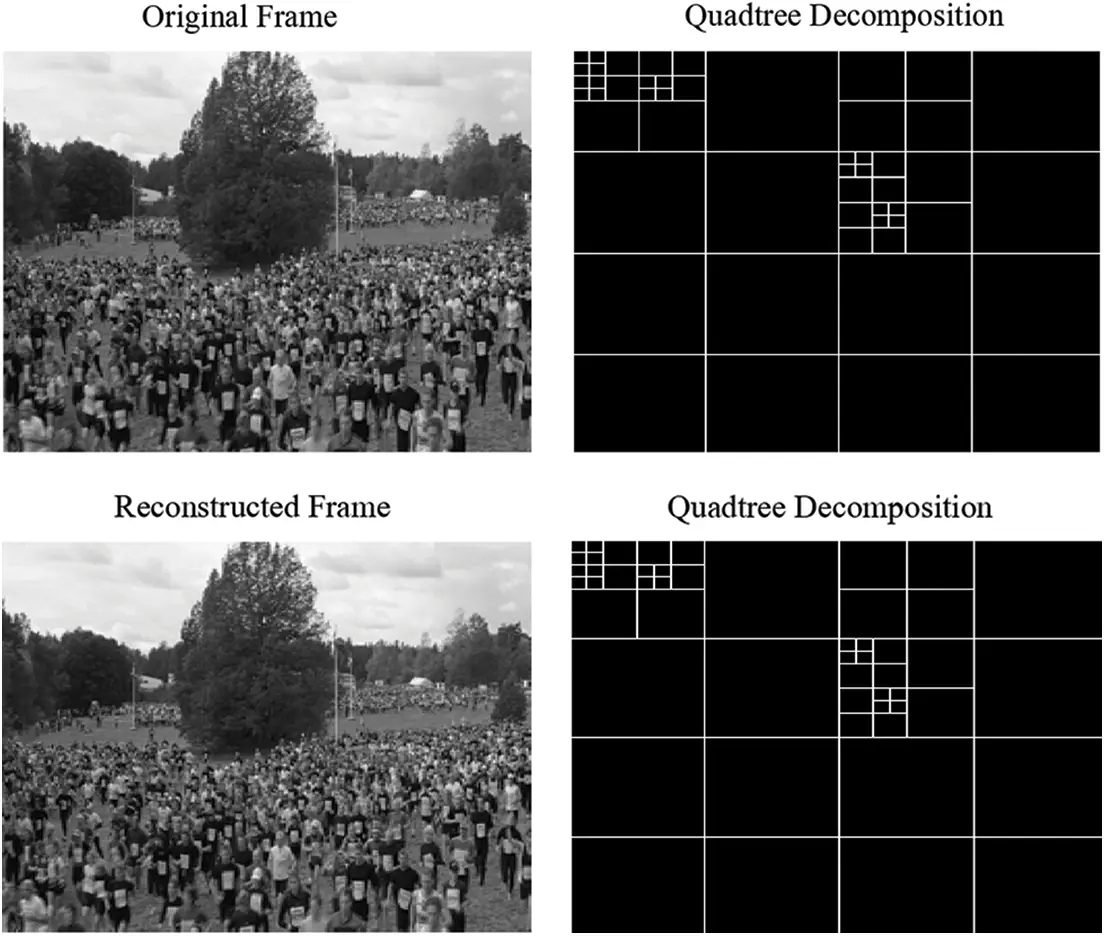Figure 5: Original and reconstructed frame with quadtree structure of optimal path (bit budget, PSNR) using AI-VQM algorithm for HD video sequences of crowd run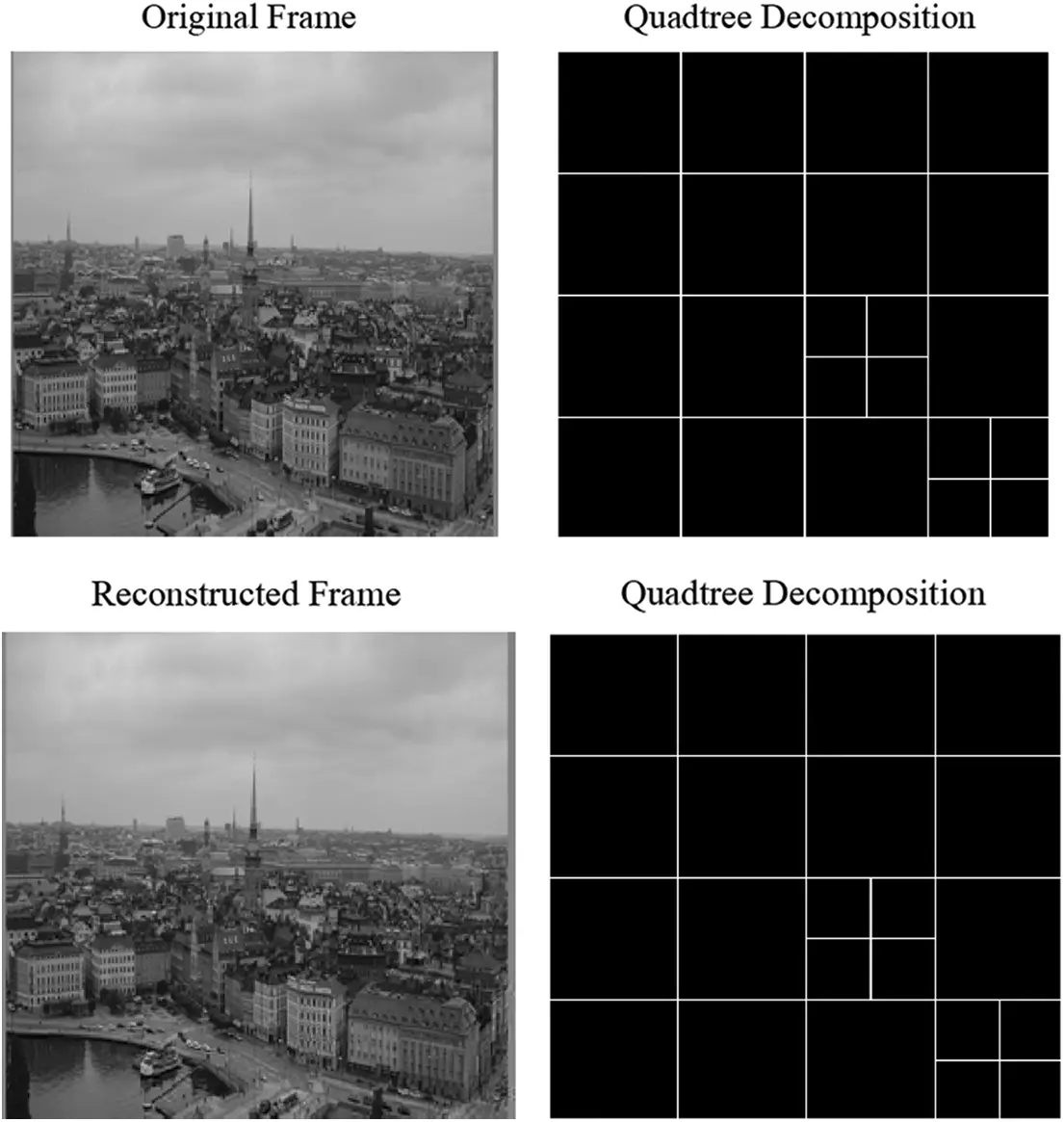Figure 6: Original and reconstructed frame with quadtree structure of optimal path (bit budget, PSNR) using the VQM algorithm for HD video sequences of the old city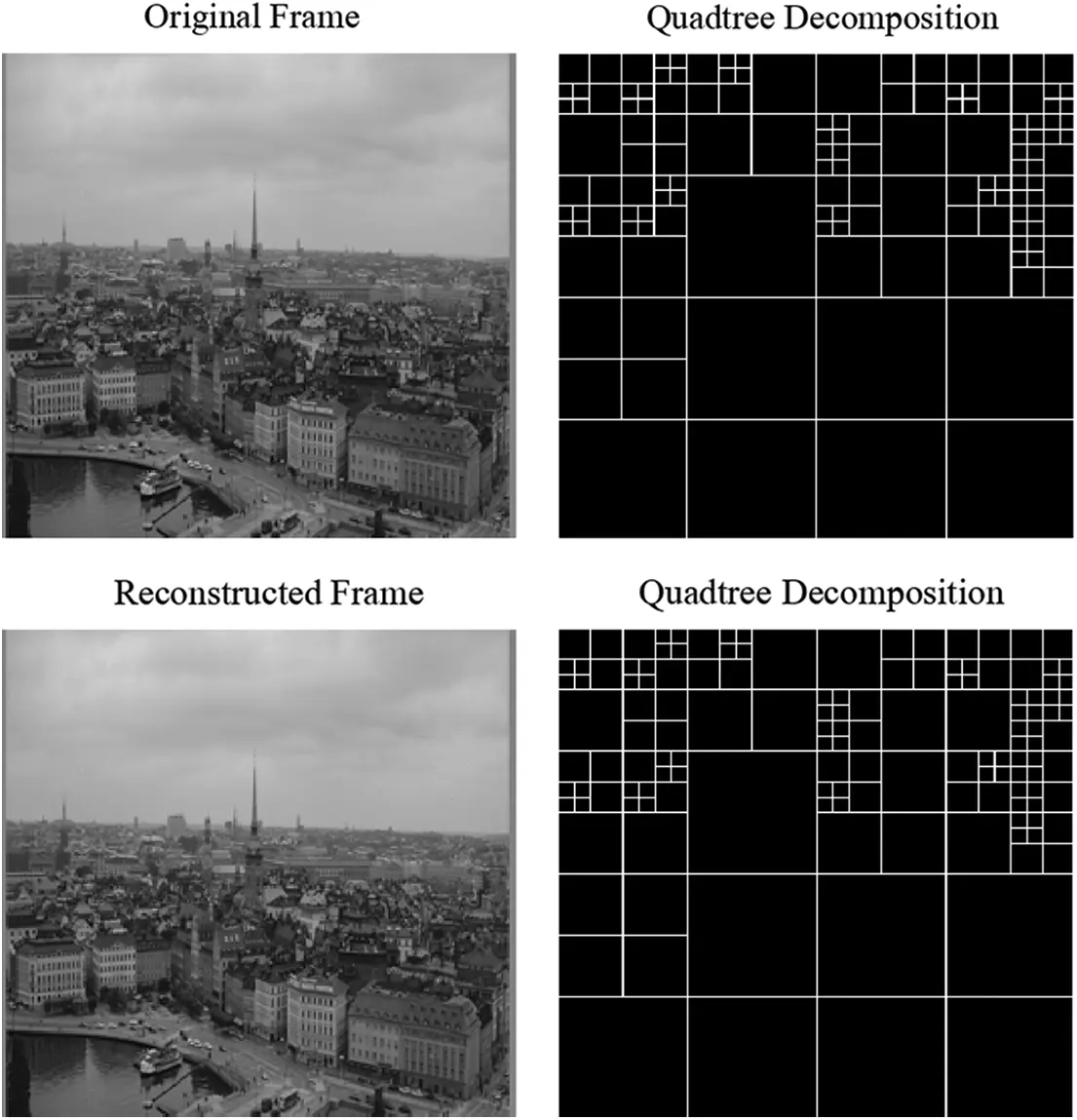Figure 7: Original and reconstructed frame with quadtree structure of most optimal path (bit budget, PSNR) using AI-VQM algorithm for HD video sequences

5  Conclusion

HEVC is the most efficient adoption of real-time, high-quality digital video content multimedia applications. In this paper, different quantization parameters are required for various classes of videos. The proposed AI-VQM has been developed for making it more feasible and efficient video sequences are achieved for multiple paths based on bit-budget, quality and reduced complexity with less loss in PSNR. Therefore, it is concluded that the proposed algorithm is more efficient than the existing algorithm in respect of different performance parameters. It is also concluded that HEVC provides the high quality of video sequence at the cost of high bit budget. In this work proposed algorithm create a balance between the bit budget and quality of video sequence.

The proposed method can be combined with different artificial intelligence and machine learning techniques for making it more efficient and computational in real-time applications. In machine learning by using compact convolution neural network and reconstruction neural network strategies may be used for high compression and high quality output video sequence. These soft computing techniques are used for quadtree generation in proposed algorithm and saving large amount of bit budget.

Funding Statement: The authors received no specific funding for this study.

Conflicts of Interest: The authors declare that they have no conflicts of interest to report regarding the present study.

## References

1. J. R. Ohm, G. J. Sullivan, H. Schwarz, T. K. Tan, and T. Wiegand, “Comparison of the coding efficiency of video coding standards—including high-efficiency video coding (HEVC),” IEEE Transaction Circuits System. Video Technology, vol. 22, no. 12, pp. 1669–1684, 2012.
2. Y. Gong, S. Wan, K. Yang, Y. Yang and B. Li, “Rate-distortion-optimization-based quantization parameter cascading technique for random-access configuration in H.265/HEVC,” IEEE Transactions on Circuits and Systems for Video Technology, vol. 27, no. 6, pp. 1304–1312, 2017.
3. G. J. Sullivan, J. -R. Ohm, W. -J. Han, and T. Wiegand, “Overview of the high-efficiency video coding standard,” IEEE Transaction Circuits System. Video Technology, vol. 22, no. 12, pp. 1648–1667, 2012.
4. D. Grois, D. Marpe, A. Mulayoff, B. Itzhaky and O. Hadar, “Performance comparison of H.265/MPEG-HEVC, VP9, and H. 264/MPEG-AVC encoders,” in Picture Coding Symp., IEEE, San-Jose, California, USA, vol. 13, no. 3, pp. 8–11, 2013.
5. X. Huang, D. Li and H. Yin, “HEVC quantization parameter selection algorithm based on inter-frame dependency,” Multimedia Tools and Application, vol. 79, no. 4, pp. 13951–13966, 2020.
6. G. Correa, P. Assuncao, L. Agostini and L. Cruz, “Complexity control of high efficiency video encoders for power-constrained devices,” IEEE Transactions on Consumer Electronics, vol. 57, no. 3, pp. 1866–1874, 2011.
7. G. Correa, P. Assuncao, L. V. Agostini and L. A. Cruz, “Motion compensated tree depth limitation for complexity control of HEVC encoding,” in IEEE Conf. on Image Processing, ICIP2012, USA, pp. 217–220, 2012.
8. F. Bossen, B. Bross, K. Sühring and D. Flynn, “HEVC complexity and implementation analysis,” IEEE Trans. Circuits System. Video Technology, vol. 22, no. 12, pp. 1684–1695, 2012.
9. D. Marpe, H. Schwarz, T. Wiegand and S. Boße, “Improved video compression technology and the tmerging high-efficiency video coding standard,” in IEEE Int. Conf. on Consumer Electronics, ICCE, Berlin, pp. 52–56, 2011.
10. P. Helle, S. Oudin, B. Bross and D. Marpe, “Block merging for quadtree-based partitioning in HEVC,” IEEE Transactions on Circuits and Systems for Video Technology, vol. 22, no. 12, pp. 1720–1731, 2012.
11. B. Lee and M. Kim, “Modelling rates and distortions based on a mixture of laplacian distributions for inter-predicted residues in quadtree coding of HEVC,” IEEE Signal Processing Letters, vol. 18, no. 10, pp. 1–15, 20
12. S. Hu, S. Kwong, T. Zhao and C. C. J. Kuo, “Rate control optimization for temporal-layer scalable video coding,” IEEE Transactions Circuits System Video Technology, vol. 21, no. 8, pp. 1152–1162, 2011.
13. H. Lee, S. Yang, Y. Park and B. Jeon, “Fast quantization method with simplified rate-distortion optimized quantization for an HEVC encoder,” IEEE Transactions on Circuits and Systems for Video Technology, vol. 26, no. 1, pp. 107–116, 2016.
14. Y. Gao, P. Liu, Y. Wu and K. Jia, “Quadtree degeneration for HEVC,” IEEE Transactions on Multimedia, vol. 18, no. 12, pp. 2321–2330, 2016.
15. X. Wang, L. Song, M. Chen and J. Yang, “Paralleling variable block size motion estimation of HEVC on CPU plus GPU platform,” in IEEE Int. Conf. on Multimedia and Expo Workshops (ICMEW), San Jose, CA, pp. 1–5, 2013.
16. K. Jaskolka and A. Kaup, “Improving the rate-distortion model of HEVC intra by integrating the maximum absolute error,” in IEEE Int. Conf. on Acoustics, Speech and Signal Processing (ICASSP), Brighton, United Kingdom, pp. 1817–1821, 2019.
17. Y. Lin and J. Lai, “Feature-based fast coding unit partition algorithm for high-efficiency video coding,” Journal of Applied Research and Technology, vol. 13, no. 2, pp. 205–219, 2015.
18. Y. Yuan, K. Kim, X. Zheng, L. Liu, X. Cao et al., “Quadtree based nonsquare block structure for inter frame coding in high efficiency video coding,” IEEE Transactions on Circuits and Systems for Video Technology, vol. 22, no. 12, pp. 1707–1719, 2012.
19. C. Wang, S. Lee and L. Chang, “Designing JPEG quantization tables based on human visual system,” Signal Processing: Image Communication, vol. 16, no. 4, pp. 501–506, 2001.
20. T. Zhao, Z. Wang and C. W. Chen, “Adaptive quantization parameter cascading in HEVC hierarchical coding,” IEEE Transaction on Image Processing, vol. 25, no. 7, pp. 2997–3009, 2016.
21. S. V. Viraktamath and G. V. Attimarad, “Impact of quantization matrix on the performance of JPEG,” International Journal of Future Generation Communication and Networking, vol. 4, no. 3, pp. 24–38, 2011.
22. J. Nam, D. Sim and I. V. Baji’c, “HEVC-Based adaptive quantization for screen content videos,” in IEEE Int. Symp. on Broadband Multimedia Systems and Broadcasting (BMSB), Seoul, Korea, pp. 1–4, 2012.
23. M. Wang, K. N. Ngan, H. Li and H. Zeng, “Improved block level adaptive quantization for high-efficiency video coding,” in IEEE Int. Symp. on Circuits and Systems (ISCAS), Lisbon, Portugal, pp. 509–512, 2015.
24. T. Nguyen, P. Helle, M. Winken, B. Bross, D. Marpe et al., “Transform coding techniques in HEVC,” IEEE Journal of Selected Topics in Signal Processing, vol. 7, no. 6, pp. 978–989, 2013.
25. R. Gweon and Y. Lee, “N-Level quantization in HEVC,” in IEEE Int. Symp. Broadband Multimedia Systems, Seoul, Korea, pp. 1–5, 2012.
26. L. Prangnell, V. Sanchez and R. Vanam “Adaptive quantization by soft-thresholding in HEVC,” in Picture Coding Symp. (PCS), Cairns, QLD, Australia, pp. 35–39, 2015.
27. Y. Chuohao, T. Hui Li and T. Yih Han, “SSIM-Based adaptive quantization in HEVC,” in IEEE Int. Conf. on Acoustics Speech and Signal Processing, Vancouver, BC, pp. 1690–1694, 2013.
28. M. Paul, A. Antony and G. Sreelekha, “Performance improvement of HEVC using adaptive quantization,” in Int. Conf. on Advances in Computing, Communications, and Informatics (ICACCI), Delhi, India, pp. 1428–143, 2014.
29. S. Im and K. Chan, “Multi-lambda search for improved rate-distortion optimization of H.265/HEVC,” in 10th Int. Conf. on Information Communications and Signal Processing (ICICS), Singapore, pp. 1–5, 2015.
30. M. Cassa, M. Naccari and F. Pereira, “Fast rate-distortion optimization for the emerging HEVC standard,” in Proc. Picture Coding Symp. (PCS), Krakow, Poland, pp. 493–496, 2012.
31. J. He and F. Yang “High-speed implementation of rate-distortion optimized quantization for H.264/AVC,” Signal Image Video Processing, vol. 9, no. 3, pp. 543–551, 2013.
32. F. Yang, Y. Zhou and J. He, “High-speed implementation of rate-distortion optimized quantization for H.265/HEVC,” IET Image Processing, vol. 9, no. 8, pp. 652–661, 2015.
33. F. Kuipers, P. V. Mieghem, T. Korkmaz and M. Krunz, “An overview of constraint-based path selection algorithms for QoS routing,” in IEEE Communications Magazine, vol. 40, no. 12, pp. 50–55, 2002.
34. G. Lu, W. Ouyang, D. Xu, X. Zhang, C. Cai et al., “DVC: An end-to-end deep video compression framework,” in IEEE Conf. on Computer Vision and Pattern Recognition (CVPR), Long Beach, CA, USA, pp. 11006–11015, 2019.
35. K. Choi, J. Chen, A. Tamse, H. Yang, M. W. Park et al., “New video codec for high-quality video service and emerging applications,” in Data Compression Conf. (DCC), Snowbird, UT, USA, pp. 310–319, 2019.
36. R. Birman, Y. Segal and O. Hadar, “Overview of research in the field of video compression using deep neural networks,” Multimedia Tools and Application, vol. 79, no. 3, pp. 11699–11722, 2020.
37. K. Kumar, R. Kumar, S. Mahajan and A. K. Pandit, “Efficiency enhancement of K-multi constraints paths in hybrid video coder using information based quantization method,” Journal Ambient Intelligent Human Computer, vol. 12, no. 2, pp. 1–15, 2021. https://doi.org/10.1007/s12652-020-02766-6.
38. R. Kumar, K. Kumar, S. Mahajan and A. K. Pandit, “Study and implementation of K-multiple constraint shortest path for H.265 HEVC for optimal video compression,” Journal Ambient Intelligent Human Computer, vol. 12, no. 2, pp. 1–16, 2021. https://doi.org/10.1007/s12652-021-03031-0.
39. W. Sun, G. Z. Dai, X. R. Zhang, X. Z. He and X. Chen, “TBE-net: A three-branch embedding network with part-aware ability and feature complementary learning for vehicle re-identification,” IEEE Transactions on Intelligent Transportation Systems, vol. 14, no. 3, pp. 1–13, 2021. https://doi.org/10.1109/TITS.2021.3130403.
40. M. Abouhawwash and A. Alessio, “Multi-objective evolutionary algorithm for PET image reconstruction: Concept,” IEEE Transactions on Medical Imaging, vol. 12, no. 4, pp. 1–10, 2021.
41. M. Abdel Basset, R. Mohamed, M. Abouhawwash, R. K. Chakrabortty and M. J. Ryan, “EA MSCA: An effective energy-aware multi-objective modified sine-cosine algorithm for real-time task scheduling in multiprocessor systems: Methods and analysis,” Expert Systems with Applications, vol. 173, no. 3, pp. 114699, 2021.
42. M. Abdel Basset, D. Elshahat, K. Deb and M. Abouhawwash, “Energy aware whale optimization algorithm for real-time task scheduling in multiprocessor systems,” Applied Soft Computing, vol. 93, no. 2, pp. 106349, 2020.
43. M. Abouhawwash, K. Deb and A. Alessio, “Exploration of multi-objective optimization with genetic algorithms for PET image reconstruction,” Journal of Nuclear Medicine, vol. 61, no. 4, pp. 572–572, 2020.This work is licensed under a Creative Commons Attribution 4.0 International License, which permits unrestricted use, distribution, and reproduction in any medium, provided the original work is properly cited.